1 / 46
• 大图
• 列表

1639

• 商品信息
• 品牌 型号 颜色 尺码
价格
预计出货日
• 购买数量
• 安大叔
• D763/C890-XXXL(荧光黄）
• 荧光黄
• XXXL
• 询价
• 有库存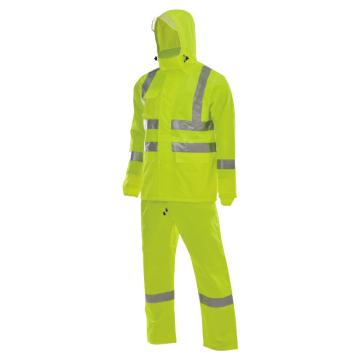- +
• 推荐
• PVC围裙-70*100
• 询价
• 有库存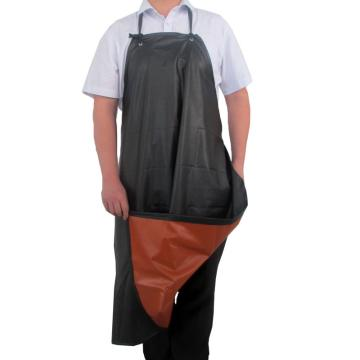- +
• 推荐
• 户外骑行雨衣
• 黑色
• XXXL
• 询价
• 有库存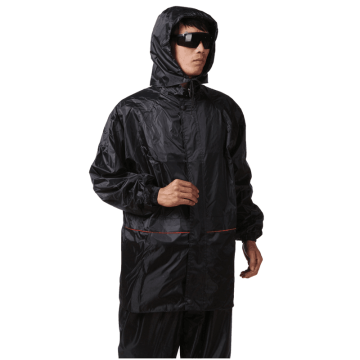- +
• PREISING
• 降温毛巾-蓝
• 蓝色
• 询价
• 有库存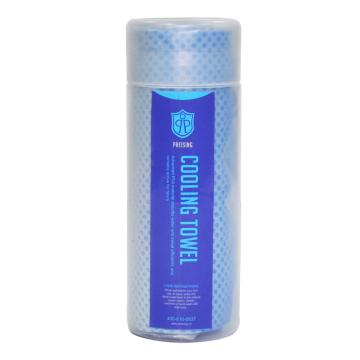- +
• 安大叔
• D766/C893-L
• 深蓝色
• L
• 询价
• 有库存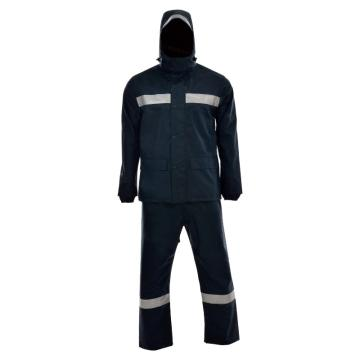- +
• 推荐
• 牛仔布围裙-60*100
• 询价
• 有库存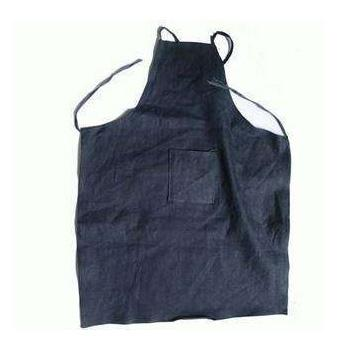- +
• 安思尔
Ansell
• 56-910-M
• 询价
• 有库存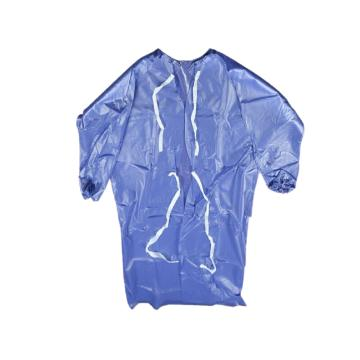- +
• 推荐
• 下水裤,尺码：41
• 黑色
• 41
• 询价
• 有库存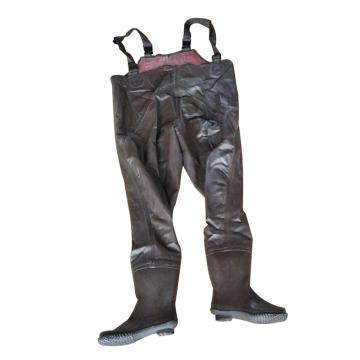- +
• TIM WORK
• 5103
• 询价
• 有库存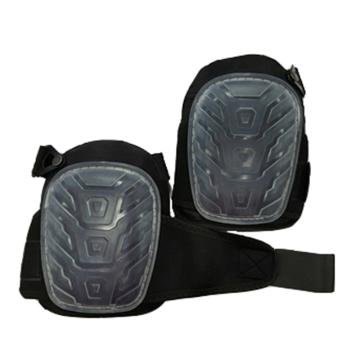- +
• TIM WORK
• 5101
• 均码
• 询价
• 有库存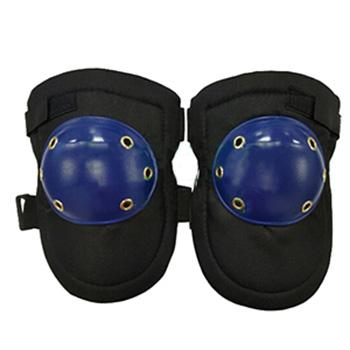- +
• 羿科
AEGLE
• 60502604-XXL
• 黄色
• XXL
• 询价
• 有库存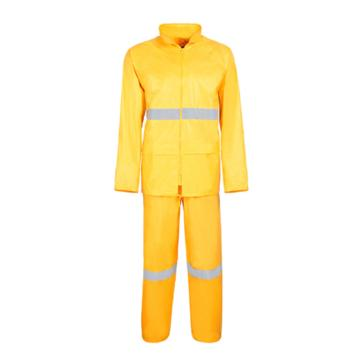- +
• 羿科
AEGLE
• 60502604-L
• 黄色
• L
• 询价
• 有库存- +
• 羿科
AEGLE
• 60502604-XL
• 黄色
• XL
• 询价
• 有库存- +
• 安大叔
• D766/C893-XL
• 深蓝色
• XL
• 询价
• 有库存- +
• 安大叔
• D763/C890-XXL(荧光黄）
• 荧光黄
• XXL
• 询价
• 有库存- +
• 安大叔
• D763/C890-XL(荧光黄）
• 荧光黄
• XL
• 询价
• 有库存- +
• 凯壹特
KIT
• 308-2（灰色）
• 灰色
• 询价
• 有库存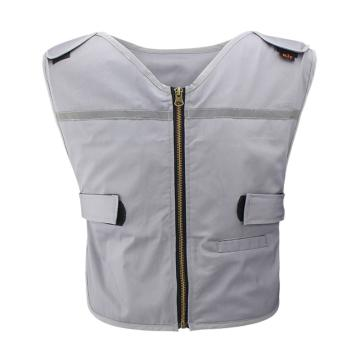- +
• 羿科
AEGLE
• 60502604-M
• 黄色
• M
• 询价
• 有库存- +
• 推荐
• 下水裤,尺码：43
• 黑色
• 43
• 询价
• 有库存- +
• 推荐
• 下水裤,尺码：42
• 黑色
• 42
• 询价
• 有库存- +
• 推荐
• 下水裤,尺码：40
• 黑色
• 40
• 询价
• 有库存- +
• 威特仕
Weldas
• 33-8200-XL
• 深蓝色
• XL
• 询价
• 有库存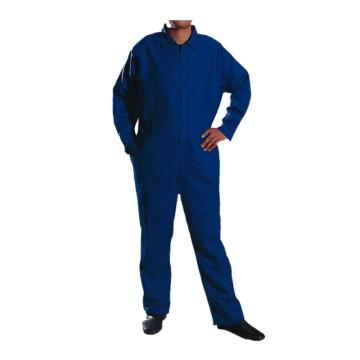- +
• 威特仕
Weldas
• 33-8200-M
• 深蓝色
• M
• 询价
• 有库存- +
• 燕王
yanwang
• 8808-L
• 白色
• L
• 询价
• 有库存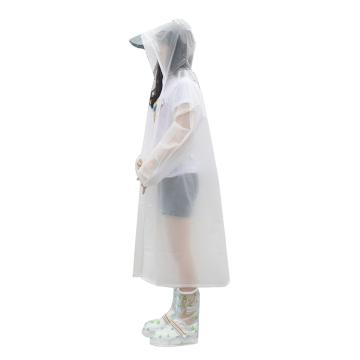- +
• 雷克兰
Lakeland
• AMN601
• 询价
• 有库存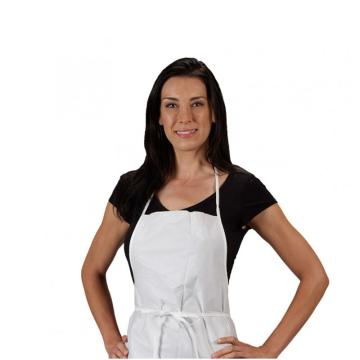- +
• 爱马斯
AMMEX
• PA1.0C
• 询价
• 有库存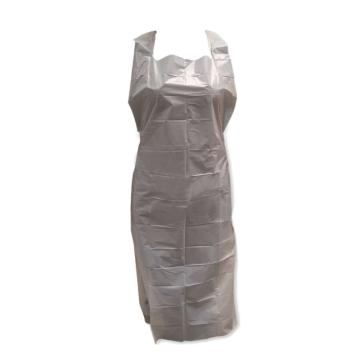- +
• 威特仕
Weldas
• 33-8200-XXXL
• 深蓝色
• XXXL
• 询价
• 有库存- +
• 沽衣邦
• 沽衣邦冬季棉连体服带反光条,深蓝色，190
• 深蓝色
• 190
• 询价
• 有库存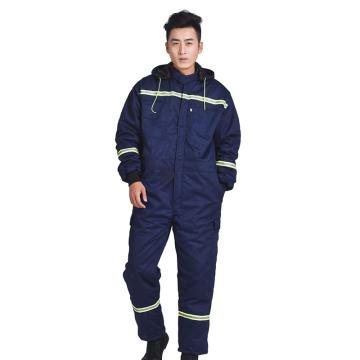- +
• 安岬
PGS
• PGS510-XL
• 深蓝色
• XL
• 询价
• 有库存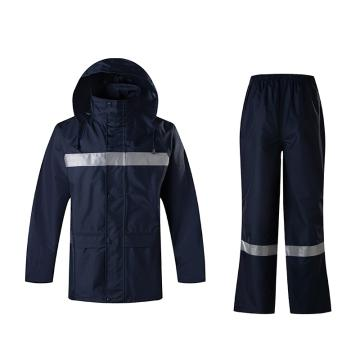- +
• 安防
ANFANG
• H003M-W175-白色
• 白色
• 175
• 询价
• 有库存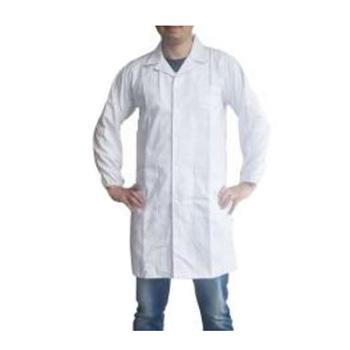- +
• 安防
ANFANG
• H003M-W170-白色
• 白色
• 170
• 询价
• 有库存- +
• 推荐
• 复合PVC防化围裙，黑色
• 询价
• 有库存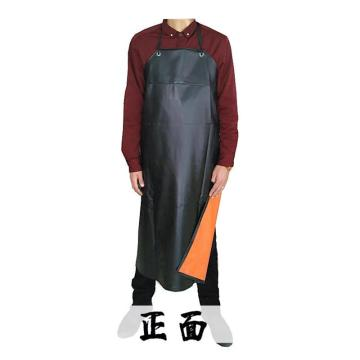- +
• 燕王
yanwang
• 8808-M
• 白色
• M
• 询价
• 有库存- +
• 锦禾
• 一次性隔离衣-XL
• 白色
• XL
• 询价
• 有库存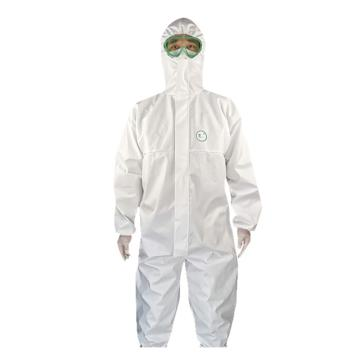- +
• 锦禾
• 一次性隔离衣-L
• 白色
• L
• 询价
• 有库存- +
• 锦禾
• 一次性隔离衣-3XL
• 白色
• 3XL
• 询价
• 有库存- +